# 5. Find the following limit and explain which limit laws you used:lim x2008 sin+ cos xr2009Dind the following limit andl ovnlein which limit lowa vou usod:lim £lo) whore £(. 5. First, we note that, for x + 0-1 < sin(+ cos x) < 1.2009Hence, for x 0, we have2008'

Question
1 views

I just need reassurance.  Because x is approaching 0 that means it cannot equal 0 but be anything greater than or equal to .  So why couldn't I say greater than 0 or less than 0?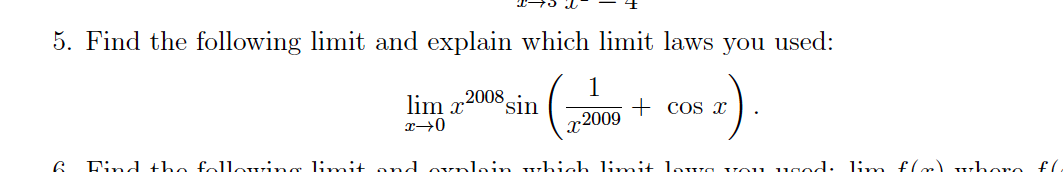help_outlineImage Transcriptionclose5. Find the following limit and explain which limit laws you used: lim x2008 sin + cos x r2009 Dind the following limit andl ovnlein which limit lowa vou usod: lim £lo) whore £(. fullscreen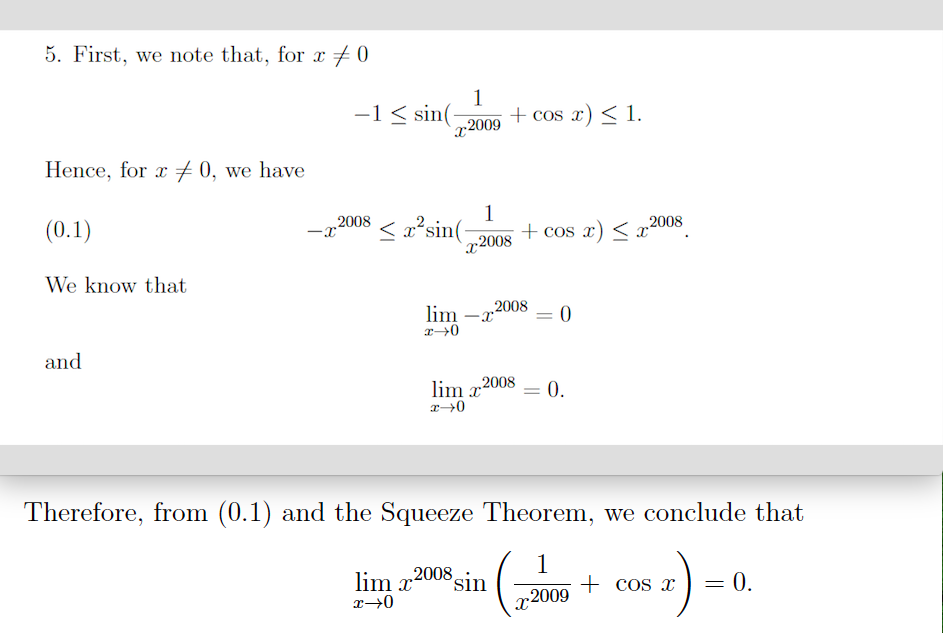help_outlineImage Transcriptionclose5. First, we note that, for x + 0 -1 < sin( + cos x) < 1. 2009 Hence, for x 0, we have 2008 '<²sin(- x2008 ,2008 (0.1) + cos x) < “ We know that 2008 lim -x and lim x2008 = 0. Therefore, from (0.1) and the Squeeze Theorem, we conclude that ( -)= 2008 sin lim x 0. + cos x -2009 fullscreen
check_circle

Step 1

Squeeze theorem states that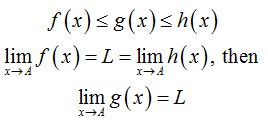Step 2

Consider,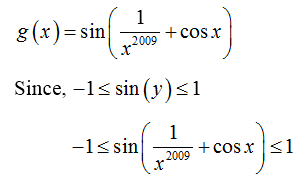Step 3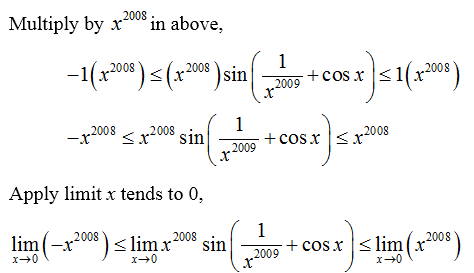...

### Want to see the full answer?

See Solution

#### Want to see this answer and more?

Solutions are written by subject experts who are available 24/7. Questions are typically answered within 1 hour.*

See Solution
*Response times may vary by subject and question.
Tagged in

### Calculus